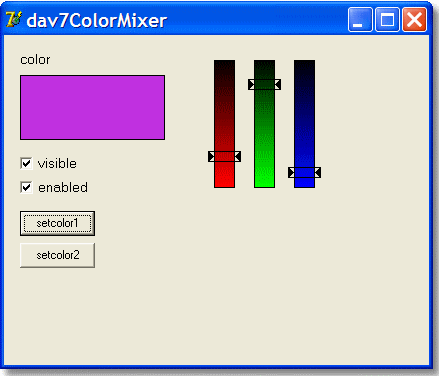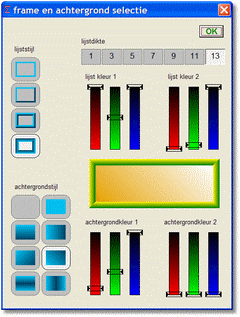a simple colormixer componentSometimes it is convenient to have a simple color selector to be placed on the canvas of a dialog form.
The component presented here is of moderate size but still capable of selecting 1 of 256000 colors, because
is is constructed of separate slides for red, green and blue, each with 64 positions.
Below is a picture of the (colormixer) component in actionThe colormixer class is placed in a separate unit.
Form1 and Unit1 make an exerciser to test the component.
The mixer only consists of the three veretical color bars.
The other components are part of the exerciser.

The only property added is "color".
By writing a new value to the color property, the slides may be preset.
Read the color property to get the selected color.
The color is always in the Windows bb-gg-rr format.
Each time a slide changes position an event is generated that presents the
new color to the application.

Each slide has a position of 0 to 63.
Before packing the slide positions into the 32 bits color value, 2 bits of value "1" are placed behind it
to make the slideposition 8 bits in length.
So, the minimal color value is \$00030303 and the highest is \$00ffffff

The colormixer component has a fixed size of 120 * 140 pixels.
Do not set the width or height.

click [ here ] to load the testprogram with the mixer.

click [ here ] to load the complete Delpi-7 project.Here you see 4 colormixers in action (picture 50% reduced) in a dialogform to select frame properties.Below is the source listing of the colormixer

```unit dav7ColorMixer;
{simple color mixer component for use on custom dialog form

DavData Software, 14 - 12 - 2011

mix r,g,b selection

property : - color

methods  : - create

events   : - OnSelect : mouse-move or mouse-down
over a color slide supplies selected color
}

interface

uses windows,controls,classes,graphics,forms;

type TColorSelect = procedure(sender : TObject; color : LongInt) of object;

Tdav7Colormixer = class(TGraphicControl)
private
Fred        : byte;    //0..63  color code cc
Fgreen      : byte;
Fblue       : byte;
FColor      : longInt;
FOnSelect   : TColorSelect;
protected
procedure paint; override;
procedure mousemove(Shift : Tshiftstate; x,y : integer); override;
procedure MouseDown(Button: TMouseButton; Shift: TShiftState;
X, Y: Integer); override;
procedure selColor(color : LongInt);
procedure clearSlide(nr : byte);
procedure setslide(nr : byte);
procedure packRGB;
procedure change(x,y : integer);
public
constructor create(AOwner:TComponent); override;
property color : longInt read FColor write selcolor;
published
property OnSelect : TcolorSelect read FOnSelect write FOnSelect;
property visible;
property enabled;
end;

procedure Register;

implementation

procedure Register;
begin
RegisterComponents('system',[Tdav7ColorMixer]);
end;

function y2color(y,nr : byte) : longInt;
//convert y slide top position to color
begin
result := (((y and \$fe) shl 1) + 3) shl ((nr-1) shl 3);
end;

procedure Tdav7ColorMixer.packRGB;
//make Fcolor from Fred,Fgreen,Fblue
begin
Fcolor := (Fred shl 2) + 3;
Fcolor := Fcolor or ((Fgreen shl 2) + 3) shl 8;
Fcolor := Fcolor or ((Fblue shl 2) + 3) shl 16;
end;

constructor Tdav7Colormixer.create(AOwner: TComponent);
begin
inherited create(Aowner);
Fred := 0;
Fgreen := 0;
Fblue := 0;
Fcolor := \$0;
width := 120;
height := 140;
end;

procedure TDav7ColorMixer.selcolor(color : LongInt);
//external call sets color of mixer
var i : byte;
begin
if (enabled = false) then exit;

Fcolor := color;
for i := 1 to 3 do
begin
if visible then clearslide(i);
case i of
1 : Fred := (color and \$ff) shr 2;
2 : Fgreen := ((color shr 8) and \$ff) shr 2;
3 : Fblue := ((color shr 16) and \$ff) shr 2;
end;
if visible then setslide(i);
end;
end;

procedure TDav7ColorMixer.clearSlide(nr : byte);
//erase slide nr
var x,y1,y2 : byte;
i,cc : byte;
begin
with self do with canvas do
begin
brush.color := getparentform(self).color;
x := (nr - 1)*40;
cc := 0;
case nr of
1 : cc := Fred;
2 : cc := Fgreen;
3 : cc := Fblue;
end;
y1 := cc shl 1;
y2 := y1 + 11;
pen.Width := 1;
pen.Color := 0;
brush.style := bsSolid;
fillrect(rect(x,y1,x+40,y2));
if y1 < 5 then y1 := 5;
if y2 > 133 then y2 := 134;
moveto(x+10,y1);
lineto(x+10,y2);
moveto(x+30,y1);
lineto(x+30,y2);
for i := 0 to 11 do
if (y1+i >= 5) and (y1+i <= 133) then
begin
if y1 + i = 133 then pen.Color := 0
else pen.Color := y2color(y1+i-5,nr);
moveto(x+11,y1+i);
lineto(x+30,y1+i);
end;//for i
end;//with
end;

procedure Tdav7ColorMixer.setslide(nr : byte);
//set slide nr acc. to bc
var x,y : byte;
i,cc : byte;
begin
cc := 0;
case nr of
1 : cc := Fred;
2 : cc := Fgreen;
3 : cc := Fblue;
end;
x := (nr-1)*40;
y := cc shl 1;
with self do with canvas do
begin
brush.style := bsClear;
pen.width := 1;
pen.color := 0;
rectangle(x+4,y,x+37,y+11);
for i := 0 to 5 do
begin
moveto(x+4+i,y+i);
lineto(x+4+i,y+11-i);
end;
x := x+36;
for i := 0 to 5 do
begin
moveto(x-i,y+i);
lineto(x-i,y+11-i);
end;
end;
end;

procedure Tdav7Colormixer.Paint;
var i,j,x1,x2,y,k : byte;
begin
with self do
with canvas do
begin
brush.color := getparentform(self).color;
pen.color := \$000000;
pen.Width := 1;
brush.style := bsSolid;
fillrect(rect(0,0,width,height));
brush.Style := bsClear;
for i := 0 to 2 do
begin
x1 := 10+40*i;
x2 := x1+21;
for j := 0 to 63 do
begin
y := 5 + 2*j;
pen.color := y2color(y-5,i+1);
for k := 0 to 1 do
begin
moveto(x1,y+k);
lineto(x2,y+k);
end;//for k
end;
pen.color := \$0;
rectangle(x1,5,x2,133);
setslide(i+1);
end;//for i
end;//with canvas
end;

procedure Tdav7ColorMixer.change(x,y: integer);
//process change
var modx,slide : byte;
proc : boolean;
begin
if (x > 0) and (x < 120) and (y > 0) and (y < 133) then
begin
modx := x mod 40;
if (modx > 5) and (modx < 35) then
begin
slide := x div 40 + 1;
y := (y - 5);
if y < 0 then y := 0
else y := y shr 1;
proc := false;
case slide of
1 : proc := Fred <> y;
2 : proc := Fgreen <> y;
3 : proc := Fblue <> y;
end;
if proc then
begin
clearslide(slide);
case slide of
1 : Fred := y;
2 : Fgreen := y;
3 : Fblue := y;
end;
setslide(slide);
packRGB;
if componentstate * [csDesigning] = [] then
if assigned(FOnSelect) then FonSelect(self,Fcolor);
end;//if proc
end;//if modx
end;//if x > 0
end;

procedure Tdav7colormixer.MouseDown(Button: TMouseButton; Shift: TShiftState;
X, Y: Integer);
begin
change(x,y);
end;

procedure Tdav7ColorMixer.mousemove(Shift : Tshiftstate; x,y : integer);
begin
if shift = [] then exit;
change(x,y);
end;

end.

```